# Bit Vectors

It should come as no surprise that the author has a fairly large collection of games. A small sampling of games, with some of their properties, is listed in Figure 12-1.

Figure 12-1. A few games from the author's collection.

(This item is displayed on page 326 in the print version)

Game

Players

Time

Difficulty

Type

Apples to Apples

48

<1 hour

low

diplomacy

Bamboleo

27

<1 hour

low

dexterity

Bohnanza

37

<1 hour

medium

diplomacy/luck

Carcassonne

25

<1 hour

medium

luck/strategy

Cosmic Wimpout

210

<1 hour

low

luck

Formula Dé

210

12 hours

medium

luck/strategy

Give Me the Brain

38

<1 hour

low

luck

El Grande

25

12 hours

high

strategy

Lord of the Fries

38

<1 hour

medium

luck

Pitchcar

28

<1 hour

low

dexterity

Puerto Rico

35

12 hours

high

strategy

Samurai Swords

25

>2 hours

high

strategy

Settlers of Catan

34

12 hours

medium

diplomacy/luck/strategy

28

>2 hours

high

strategy

Twister

24

<1 hour

low

dexterity

When the author gets together with friends, he often has to answer the question, "Which game shall we play?" Sometimes people want something quick and light that can be played while waiting for others to arrive. Other times, people are ready to settle down for an evening-long brain-burner. If, for example, there are five people and they want to play a strategy game taking 12 hours, the options are Formula Dé, El Grande, and Puerto Rico. Let's write a program to list the appropriate games for any situation.

We could maintain a set of Game objects, each of which has a field for each of the various attributes. A more space-efficient option is to maintain, for each game, a single integer encoding all of the game's attributes (except the title). This representation is called a bit vector (Figure 12-2).

Figure 12-2. In a bit vector, each bit represents a single feature of the game. For example, El Grande can take 2, 3, 4, or 5, players, plays in 12 hours, is of high difficulty, and involves strategy.

(This item is displayed on page 327 in the print version)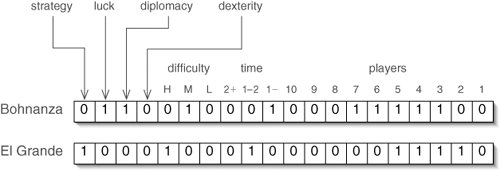If we think of each game as having a set of features, we recognize this as a variation of direct addressing (Section 11.4). For example, the bit vector for Bohnanza represent the set of features:

{3-player, 4-player, 5-player, 6-player, 7-player, less-than-1-hour, medium-difficulty, diplomacy, luck}

Bit vectors make it easy to efficiently perform certain set operations, such as intersection and union. For example, if we want to know what Bohnanza and El Grande have in common, we take the intersection of their feature sets (Figure 12-3).

Figure 12-3. The bitwise intersection of two bit vectors tells what elements two sets have in common. In the resulting bit vector, the bits that are on in both of the others are on. Here, Bohnanza and El Grande can both handle 35 players.

(This item is displayed on page 327 in the print version)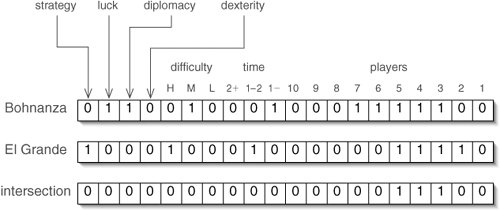If we want to know if a game is suitable for a particular situation, we can make up a bit vector for the situation (Figure 12-4). The intersection of a game's bit vector with the situation bit vector with the situation bit vector tells what they have in common. If this is equal to the situation bit vector, the game has all of the required features.

Figure 12-4. If a game's intersection with the situation equals the situation, the game is suitable. It does not matter that this situation does not specify a desired difficulty level.

(This item is displayed on page 327 in the print version)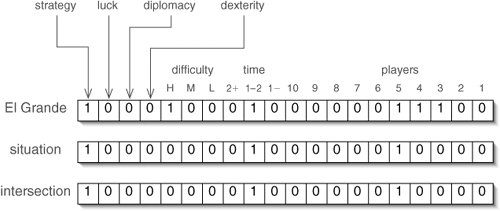In Java, we can represent a bit vector with up to 32 features using an int. The bit vector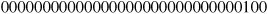represents the number 4 in binary. In our example, this bit vector also represents a 3-player game, with the extra bits at the left being ignored.

In binary, the number 2i is represented by a bit vector with only the ith bit from the right turned on. Put another way, it is a 1 with i zeroes after it.

Java has an operator << for shifting a pattern of bits to the left a given number of spaces. If we want a bit vector with only the fifth bit turned on, we use the Java expression:

```1 << 5
```

If we want several bits turned on, we simply take the union of the bit vectors for the individual bits. The bitwise or operator | allows us to find the union of two bit vectors. For example, to produce the bit vector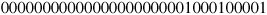we use the Java expression:

```(1 << 0) | (1 << 5) | (1 << 9)
```

Manipulating individual bits like this manually would be incredibly tedious and error prone. Instead, we define constants (Figure 12-5). The static method playerRange() is provided because many games can accept a range of player numbers.

Figure 12-5. Constants and the playerRange() function make specifying bit vectors for games much easier.

 ``` 1 /** A game with this feature takes less than an hour to play. */ 2 public static final int LESS_THAN_ONE_HOUR = 1 << 10; 3 4 /** A game with this feature takes an hour or two to play. */ 5 public static final int ONE_TO_TWO_HOURS = 1 << 11; 6 7 /** A game with this feature takes over two hours to play. */ 8 public static final int OVER_TWO_HOURS = 1 << 12; 9 10 /** A game with this feature is easy to pick up. */ 11 public static final int LOW_DIFFICULTY = 1 << 13; 12 ``` 13 /** A game with this feature is of moderate difficulty. */ 14 public static final int MEDIUM_DIFFICULTY = 1 << 14; 15 16 /** A game with this feature take considerable study to play. */ 17 public static final int HIGH_DIFFICULTY = 1 << 15; 18 19 /** A game with this feature involves agility or a steady hand. */ 20 public static final int DEXTERITY = 1 << 16; 21 22 /** A game with this feature involves talking people into things. */ 23 public static final int DIPLOMACY = 1 << 17; 24 25 /** A game with this feature involves significant randomness. */ 26 public static final int LUCK = 1 << 18; 27 28 /** A game with this feature involves careful planning. */ 29 public static final int STRATEGY = 1 << 19; 30 31 /** 32 * Return a bit vector with a feature for each number of players 33 * from minPlayers through maxPlayers. 34 */ 35 public static int playerRange(int minPlayers, int maxPlayers) { 36 int result = 0; 37 for (int i = minPlayers; i <= maxPlayers; i++) { 38 result |= 1 << (i - 1); 39 } 40 return result; 41 }

Now we can specify the bit vector for Lord of the Fries simply as:

```playerRange(3, 8) | LESS_THAN_AN_HOUR | MEDIUM_DIFFICULTY | LUCK
```

Java's bitwise operators are listed in Figure 12-6. Almost all modern processors have built-in instructions for these operations, so they are extremely fast.

Figure 12-6. Bitwise operators. Assignment operators such as & = and << = are also available.

(This item is displayed on page 330 in the print version)

Operator

Description

Notes

&

bitwise and

result is on where both operands are on for taking intersections

|

bitwise or

result is on where at least one operand is on for taking unions

^

bitwise exclusive or (xor)

result is on where exactly one operand is on

~

bitwise not

unary result is on where operand is off

<<

shift left

shifts in zero from right

>>

shift right

copies leftmost bit use with numbers

>>>

shift right

shifts in zero from left use with bit vectors

Figure 12-7 provides some examples of these operations.

Figure 12-7. Examples of bitwise operations. The values of a and b are arbitrary.

(This item is displayed on page 330 in the print version)

Expression

Bit Vector

a

10000000101010101010101000000000

b

00000000110011001100110000000000

a & b

00000000100010001000100000000000

a | b

10000000111011101110111000000000

a ^ b

10000000011001100110011000000000

~a

01111111010101010101010111111111

a << 3

00000101010101010101000000000000

a >> 3

11110000000101010101010101000000

a >>> 3

00010000000101010101010101000000

A couple of things to watch out for:

• Be careful not to confuse the logical and operator && with the bitwise and operator &. The former is used with boolean values, the latter with numerical primitive types. For added confusion, if you use & on two boolean values, you'll get their logical andbut not short-circuited! A similar warning applies to || vs |.
• There are two different shift right operators, which differ in the way they handle the leftmost bit. Suppose we have the bit vector:

```10000000010000000000001000000000
```

Shifting it two places to the right with >>> does what we would expect:

```00100000000100000000000010000000
```

In contrast, shifting it two places to the right with >> copies the leftmost bit:

```11100000000100000000000010000000
```

This option is included because bitwise operators are also sometimes used to multiply and divide ints by powers of two. In decimal notation, we can multiply a number by 103 = 1,000 by shifting it three places left. Similarly, in binary, we can multiply a number by 23 = 8 by shifting it three places left. To divide by a power of two, we shift to the right.

Computers represent negative integers using a special binary notation which is beyond the scope of this book. The important detail here is that the leftmost bit is a 1 in a negative number, so shifting a negative number to the right with >>> would incorrectly produce a positive result. The >> works correctly for division.

We should use >> for numerical division by powers of two, but >>> for shifting bit vectors to the right.

We now know more than enough to write the GameCollection class (Figure 12-8). The only nonconstant field is games, which maps Strings (game titles) to Integers (bit vectors).

Figure 12-8. The GameCollection class.

 1 import java.util.Map; 2 import java.util.TreeMap; 3 4 /** A bunch of games and their associated attributes. */ 5 public class GameCollection { 6 7 // See Figure 12-5 for constants 8 9 /** Map associating game titles with attribute bit vectors. */ 10 private Map games; 11 12 /** A GameCollection is initially empty. */ 13 public GameCollection() { 14 games = new TreeMap(); 15 } 16 17 /** Add a new game to this collection. */ 18 public void addGame(String title, int attributes) { 19 games.put(title, attributes); 20 } 21 22 /** 23 * Print the names of games which have all of the features in the 24 * constraints bit vector. 25 */ 26 public void findGames(int constraints) { 27 for (Map.Entry game : games.entrySet()) { 28 if ((constraints & game.getValue()) == constraints) { 29 System.out.println(game.getKey()); 30 } 31 } 32 } 33 34 // See Figure 12-5 for the playerRange() method 35 36 }

The loop on lines 2731 iterates through the entries in this map. Each value of game is of type Map.Entry, so we can extract the key (title) or value (attribute bit vector) of the entry as needed.

A main() method which adds all of the games in Figure 12-1 and then invokes findGames() is shown in Figure 12-9.

Figure 12-9. After adding a bunch of games to the database, we can ask for one fitting certain constraints.

 ``` 1 /** Create a GameCollection, fill it, and find some for today. */ 2 public static void main(String[] args) { 3 GameCollection collection = new GameCollection(); 4 collection.addGame("Apples to Apples", 5 playerRange(4, 8) | LESS_THAN_ONE_HOUR 6 | LOW_DIFFICULTY | DIPLOMACY); 7 collection.addGame("Bamboleo", 8 playerRange(2, 7) | LESS_THAN_ONE_HOUR 9 | LOW_DIFFICULTY | DEXTERITY); 10 collection.addGame("Bohnanza", 11 playerRange(3, 7) | LESS_THAN_ONE_HOUR 12 | MEDIUM_DIFFICULTY | DIPLOMACY | LUCK); 13 collection.addGame("Carcassonne", 14 playerRange(2, 5) | LESS_THAN_ONE_HOUR 15 | MEDIUM_DIFFICULTY | LUCK | STRATEGY); ``` 16 collection.addGame("Cosmic Wimpout", 17 playerRange(2, 10) | LESS_THAN_ONE_HOUR 18 | LOW_DIFFICULTY | LUCK); 19 collection.addGame("Formula De", 20 playerRange(2, 10) | ONE_TO_TWO_HOURS 21 | MEDIUM_DIFFICULTY | LUCK | STRATEGY); 22 collection.addGame("Give Me the Brain", 23 playerRange(3, 8) | LESS_THAN_ONE_HOUR 24 | LOW_DIFFICULTY | LUCK); 25 collection.addGame("El Grande", 26 playerRange(2, 5) | ONE_TO_TWO_HOURS 27 | HIGH_DIFFICULTY | STRATEGY); 28 collection.addGame("Lord of the Fries", 29 playerRange(3, 8) | LESS_THAN_ONE_HOUR 30 | MEDIUM_DIFFICULTY | LUCK); 31 collection.addGame("Pitchcar", 32 playerRange(2, 8) | LESS_THAN_ONE_HOUR 33 | LOW_DIFFICULTY | DEXTERITY); 34 collection.addGame("Puerto Rico", 35 playerRange(3, 5) | ONE_TO_TWO_HOURS 36 | HIGH_DIFFICULTY | STRATEGY); 37 collection.addGame("Samurai Swords", 38 playerRange(2, 5) | OVER_TWO_HOURS 39 | HIGH_DIFFICULTY | STRATEGY); 40 collection.addGame("Settlers of Catan", 41 playerRange(3, 4) | ONE_TO_TWO_HOURS 42 | MEDIUM_DIFFICULTY | DIPLOMACY | LUCK 43 | STRATEGY); 44 collection.addGame("Starmada", 45 playerRange(2, 8) | OVER_TWO_HOURS 46 | HIGH_DIFFICULTY | STRATEGY); 47 collection.addGame("Twister", 48 playerRange(2, 4) | LESS_THAN_ONE_HOUR 49 | LOW_DIFFICULTY | DEXTERITY); 50 collection.findGames(playerRange(5, 5) | ONE_TO_TWO_HOURS 51 | STRATEGY); 52

BitSets

If we want to keep track of a set with more than 32 potential elements, we can use the BitSet class in the java.util package (Figure 12-10). A BitSet represents a long bit vector as a series of binary numbers. It performs arithmetic (similar to that we'll do in Section 12.3) to find the right bit in the right number. Like an ArrayList, a BitSet can also grow as necessary. Of course, since BitSet is an encapsulated class, we don't have to think about the details; we can simply treat it as an arbitrarily long bit vector.

Figure 12-10. UML class diagram showing some of the methods in the java.util.BitSet class.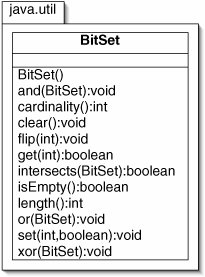The and(), or(), and xor() methods have return types of void. Rather than returning a new BitSet, each of these modifies the existing BitSet. For example, if a is the BitSet 101100 and b is the BitSet 1010, then invoking a.or(b) changes a to 101110.

The cardinality() method returns the number of bits in a BitSet which are on. In contrast, length() returns the number of bits which are "in use," ignoring any leading zeroes. Continuing the example above, b.cardinality() returns 2, but b.length() returns 4.

The other methods are self-explanatory, given that int arguments specify indices in the BitSet. See the API for additional details and a few other methods.

Exercises

 12.1 What is the value of 23 & 17? 12.2 What is the value of 23 | 17? 12.3 What is the value of 23 ^ 17? 12.4 What is the value of 23 << 5? 12.5 What is the value of 23 >> 2? 12.6 Give an expression that returns true if and only if the int n represents an empty bit vector. 12.7 Give an expression that returns true if and only if bit i of int n is on. 12.8 Given an int representation of a game, write an expression that returns true if and only if the game does not involve luck. 12.9 Speculate on why the player numbers in Figure 12-2 increase from right to left rather than left to right. 12.10 The GameCollection class uses a TreeMap. A HashMap would also work. Why would a HashMap be less efficient? 12.11 There are &= and |= operators, but there is no ~= operator. Why? (Hint: Try using ~= in a meaningful expression.) 12.12 Discuss whether the bitwise operators, such as &, are short-circuited. 12.13 Given two values a and b, a xor b is true when exactly one of a or b is true. The bitwise xor operator is ^. How would you find the logical xor of two boolean values?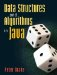Data Structures and Algorithms in Java
ISBN: 0131469142
EAN: 2147483647
Year: 2004
Pages: 216
Authors: Peter Drake